Reach Us+44-1522-440391
Lie Group Analysis for Solving the Problem of Diffusion of Drugs across a Biological Membrane | OMICS International
Journal of Generalized Lie Theory and Applications
All submissions of the EM system will be redirected to Online Manuscript Submission System. Authors are requested to submit articles directly to Online Manuscript Submission System of respective journal.

Lie Group Analysis for Solving the Problem of Diffusion of Drugs across a Biological Membrane

Abd-el-Malek MB1,2* and Amin AM1

1Department of Engineering Mathematics and Physics, Alexandria University, Alexandria 21544, Egypt

2Department of Mathematics and Actuarial Science, The American University in Cairo, New Cairo 11835, Egypt

Corresponding Author:
Abd-el-Malek MB
Department of Engineering Mathematics and Physics
Faculty of Engineering, Alexandria University
Alexandria 21544, Egypt,
Tel: 01000066496
E-mail: [email protected]

Received date: August 10, 2015; Accepted date: November 20, 2015; Published date: November 25, 2015

Citation: Abd-el-Malek MB, Amin AM (2015) Lie Group Analysis for Solving the Problem of Diffusion of Drugs across a Biological Membrane. J Generalized Lie Theory Appl 9:236. doi:10.4172/1736-4337.1000236

Copyright: © 2015 Abd-el-Malek MB, et al. This is an open-access article distributed under the terms of the Creative Commons Attribution License, which permits unrestricted use, distribution, and reproduction in any medium, provided the original author and source are credited.

Visit for more related articles at Journal of Generalized Lie Theory and Applications

Abstract

The Lie group method is applied to study the diffusion process of drugs across a biological membrane which tends to partially absorb the drug. For the diffusion coefficient, we considered two cases. The Lie group analysis is based on reducing the number of independent variables by one, and consequently the mathematical model described by nonlinear partial differential equation to, covers the diffusion process with the boundary and initial conditions, and is transformed into an ordinary differential equation with the corresponding conditions. The obtained nonlinear ordinary differential equation is solved numerically using the 4th and 5th Runge Kutta method, and the results are illustrated graphically and in tables too.

Keywords

Biological membrane; Diffusion of drugs; Concentration of drug; Absorption

Introduction

The diffusion of drugs across a composite structure such as a biological membrane is one of the interesting types of diffusion studies and is of great importance in the pharmaceutical sciences [1-4]. In 2002, Abd-el-Malek et al.  considered the problem of diffusion of a drug through a thin membrane to define the concentration of drug in the membrane in addition to that in the donor and the receiver cells, by applying the group theoretic approach which is a special case of the Lie group analysis and leads to limited results.

Several experiments were carried out to study such diffusion process, one of such experiments was carried out by Hoogervorst et al.  and Spacek and Kubin .

Spoelstra and Van Wyk  described the process as it is modeled by two cells of the same volume, called donor and the receiver cells, which are separated by a thin membrane. In the donor cell a high concentration of a drug in a saline solution is placed and the receiver cell contains only a saline solution. Initially, the membrane is free from the drug, the two cells are stirred continually, and the drug starts to diffuse through the membrane. The concentration of drug in the receiver cell and also in the membrane begins to increase. They solved the problem numerically using the finite-difference method only to evaluate the values of the parameters of the model and to define the concentration in the donor and the receiver cells.

Here we apply the Lie group analysis, which is a powerful and fundamental tool that provides invariant solutions to boundary value problems. It is applicable to both linear and nonlinear differential equations. The mathematical technique we use is one-parameter group transformation. The Lie group analysis expresses the infinitesimals of a group in terms of one or more functions, called infinitesimal functions, each of which depends on independent and dependent variables. The procedure for finding the infinitesimals is then reduced to finding the auxiliary equation. This can be obtained by solving set of partial differential equations that arise as a result of invoking invariance of the partial differential equation and its auxiliary conditions. Hence, the Lie group analysis can be used to solve a wider variety of nonlinear problems.

Mathematical formulation

The following assumptions are considered to construct our model:

(1) The rate of absorption at any point in the membrane is proportional to the concentration of the drug at the point,

(2) The x-axis of the coordinate system is taken in the direction of the normal on the membrane,

(3) No diffusion takes place through the edges of the membrane,

(4) The concentration in the membrane is considered as a function of time and of the x-coordinate, and

(5) The rate of transfer of diffusion in the membrane, per unit area, is proportional to the gradient of the concentration across the membrane.

Let C(x, t) be the concentration of the drug at a distance "x" from one face of the membrane and at an instant of time "t" and P(x, t) be the function which determines the coefficient of diffusion. The concentrations of drug in the donor and the receiver cells are D(t) and R(t), respectively.

Consider a membrane of unit thickness and if "Q" is the coefficient of partition, "q" is the coefficient which determines the rate of absorption of the drug in the membrane.

The equation which governs the diffusion process may be written as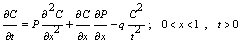, (2.1)

with the following conditions.

(i) Initial condition: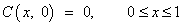(2.2)

(ii) Boundary conditions: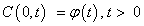(2.3)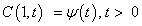(2.4)

Where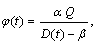(2.5)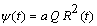(2.6)

Lie symmetry group method

According to Lie’s method [9-11], the infinitesimal generator of the symmetry group admitted by (2.1) is given by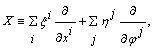(2.7)

where

i = 1, 2, j = 1, 2, and xi is independent variable (x, t) and φj is dependent variable (C, P).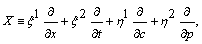(2.8)

in which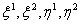are infinitesimal functions of the group variables. Then, the corresponding one-parameter Lie group of transformations is given by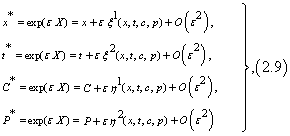If (2.9) is left invariant by the transformation then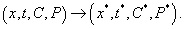Equivalently, we can obtain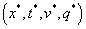by solving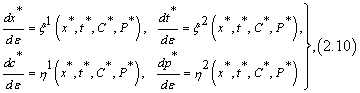subject to initial condition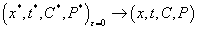Since the equation which governs the diffusion has at most second – order derivatives, the second prolongation of the generator should be considered in the form: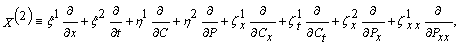(2.11)

where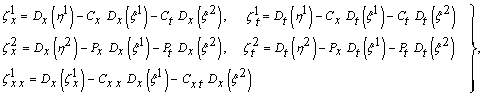(2.12)

A vector X given by (2.11) is said to be a Lie point symmetry vector field for equations (2.1) with Invariant condition X(2){2.1} = 0, and solving this system in view of the invariance of the initial and boundary conditions we get the determining equation: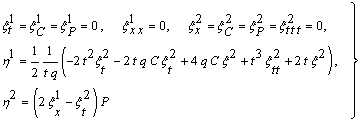(2.13)

Solving we get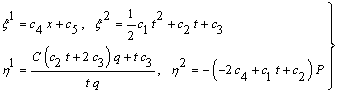(2.14)

The auxiliary equation will be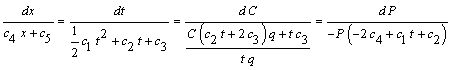(2.15)

According to our initial and boundary condition we found the solution when c2 ≠ 0 and all others will be zeros and substitute in (2.15) we get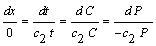(2.16)

Solving we get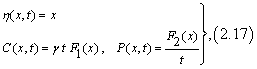where γ is an arbitrary constant and F1(x) and F2(x) are arbitrary function of x.

By substituting (2.17) in (2.1), we get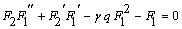(2.18)

and if we consider:

F1(0) = α Q, and substitute in (2.3) and (2.5) we get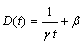(2.19)

F1(1) = Q, and substitute in (2.4) and (2.6) we get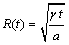(2.20)

Now consider the following two cases for F2(x):

Case (1): F2(x) = e-x and substitute in (2.18) we get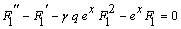(2.21)

With boundary condition

F1(0) = α Q (2.22)

F1(1) = Q (2.23)

From the results of Figure 1, it is clear that the concentration C(x, t) is slowly decreasing from the initial border of the membrane to the other.

Figure 1: Effect of time on the concentration C(x, t) through the membrane for α = 2, Q = 81.95,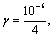q = 1.16.

From Figure 2, it is clear that the concentration in the donor cell D(t) decreases with time and the concentration in the receiving cell R(t) increases time which is compatible with our physical model.

Figure 2: Concentration of the donor and the receiver cells for β= 40000,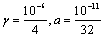.

From Figure 3, it is clear that the concentration C(x, t) in the membrane is increasing with time since our membrane does not contain any drug but at the same time the points near to initial border has higher concentration.

Figure 3: Concentration C(x, t) through the membrane for α = 2, Q = 81.95,q = 1.16.

From Table 1, it is clear that the concentration C(x, t) is slowly decreasing with distance and is increasing with time.

x F1(x) C (x, 50) C (x, 150)
0.0 163.9000 0.00204875 0.00614625
0.1 152.0091 0.00190011 0.00570034
0.2 140.6348 0.00175794 0.00527380
0.3 129.8698 0.00162337 0.00487012
0.4 119.8154 0.00149769 0.00449308
0.5 110.5853 0.00138232 0.00414695
0.6 102.2979 0.00127872 0.00383617
0.7 095.1040 0.00118880 0.00356640
0.8 089.1688 0.00111461 0.00334383
0.9 084.6975 0.00105872 0.00317616
1.0 081.9500 0.00102438 0.00307312

Table 1: F1(x), C (x, 50) and C (x, 150) for α = 2, Q = 81.95,q = 1.16.

Case (2):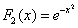, and substitute in (2.18) we get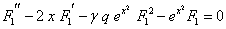(2.24)

with boundary condition

F1(0) = α Q (2.25)

F1(1) = Q (2.26)

From Figure 4, we notice that the concentration C(x, t) is decreasing from the initial border of the membrane to the other border.

Figure 4: Effect of time on the concentration C(x, t) through the membrane for α = 2, Q = 81.95,q = 1.16.

In Figure 5, we studied effect of time on the concentration; we found that firstly the concentration C(x, t) in the membrane increased with time since our membrane does not contain any drug, but at the same time the points near to the initial border has higher concentration.

Figure 5: Effect of time on the concentration C(x, t) through the membrane for α = 2, Q = 81.95,q = 1.16.

Table 2, illustrates the concentration C(x, t) is slowly decreasing with distance and is increasing with time.

x F1(x) C (x, 50) C (x, 150)
0.0 163.9000 0.00204875 0.00614625
0.1 151.5883 0.00189485 0.00568456
0.2 140.5958 0.00175745 0.00527234
0.3 130.6503 0.00163313 0.00489939
0.4 121.5661 0.00151958 0.00455873
0.5 113.2246 0.00141531 0.00424592
0.6 105.5353 0.00131919 0.00395757
0.7 098.4788 0.00123098 0.00369296
0.8 092.0940 0.00115118 0.00345352
0.9 086.5033 0.00108129 0.00324387
1.0 081.9500 0.00102438 0.00307312

Table 2 : F1(x), C (x, 50) and C (x, 150) for α = 2, Q = 81.95,q = 1.16.

The relative difference between two cases: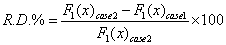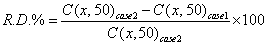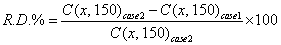We find R.D% in three cases typical as below

Table 3 illustrates that, the small relative variation in concentration for the two cases is explained in Tables 1 and 2, therefore the effect of the diffusion coefficient in Eq. (2.1) very small (Figure 6).

 x 0 0.1 0.2 0.3 0.4 0.5 0.6 0.7 0.8 0.9 1 R.D.% 0 - 0.28 - 0.03 0.6 1.44 2.33 3.07 3.43 3.18 2.09 0

Table 3: Illustrates R.D%.

The value of R.D.% increases gradually against x and reached to its maximum value at x = 0.7, then starts to decrease again and reaches zero at x = 1.

Conclusion

Non-linear partial differential equation, has been solved using Lie group analysis, and C(x, t) and P(x, t) have been evaluated analytically using the parameters of an experimental Study . The results obtained map the state of the membrane in a way similar to the one obtained by Spoelstra and van Wyk , using a finite-difference method.

The other transformations, like spiral transform and linear transform, do not work for leaving the differential equation as well as the initial and boundary conditions invariant.

The concentration proportional linear with time and P(x, t) which describes the coefficient of diffusion is inversely proportional with time. Value of R.D.% depends on the method of numerical solution to equation (2.18) and the value of F1(x).

References

Select your language of interest to view the total content in your interested language

Article Usage

• Total views: 9063
• [From(publication date):
December-2015 - Jun 17, 2019]
• Breakdown by view type
• HTML page views : 8826Can't read the image? click here to refresh